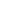TextWidth FunctionCalculate the width of a string of text.Syntax

 [C#] `int TextWidth(string text)` [Visual Basic] `Function TextWidth(text As String) As Integer`Params

 Name Description text The text return The width of the text in 1000ths.Notes

 This function caculates the width for a given text string. The value returned is measured in thousandths of a unit. So to calculate the physical size on the page multiply the returned value by the font size and divide by one thousand.Example

 None.